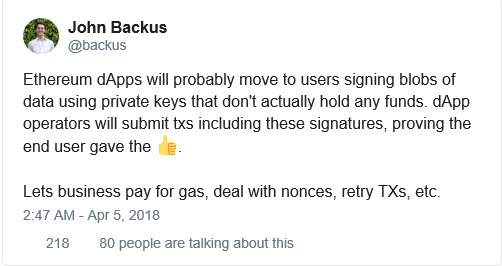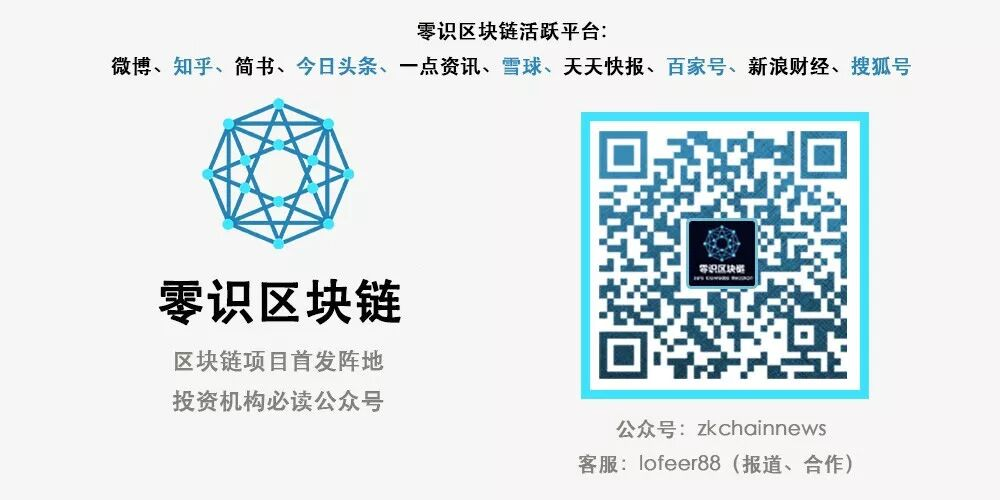# 用户交易免gas？这里有良方！1、简要概述了公钥密码学及数字签名，这是理解这项解决方案的关键。

2、解决方案详情及新应用程序流

3、实施细节（前端js与Solidity合约的代码）

4、对潜在的问题及强化措施进行论述Pu = “0x44ac12c1e3dfd8edaf83b6f65918229d5279a6f5”

Pr = “dbc226043e390cf39280e5edfd418d7ad61931c76509270867d300f110c46506”

signature = 0x9127112de0033555c7f6508d963d484965a953844dfcff092712102c236467a25af57edc53b63880ea39af8ce7334f6d77a8206e805305e7c6ad919d12bfae5c1b1. 投票人通过用自己的私钥为信息添加签名来表明他们为某个候选人投票的意愿。他们不会将其交易提交到区块链，故而不必支付任何gas。上图中的信息队列只是链下位置，所有的投票细节都存储在这里。
2. 任何愿意支付税费（通常是合约的所有者）的人可以获取签名、候选人姓名和投票人账户地址，并将其提交到区块链上。
3. 智能合约基于候选人姓名和签名利用验证函数衍生出公钥（以太坊账户地址）。如果衍生出的公钥与给消息添加签名的用户地址一致，智能合约就会记录下这次投票，否则将拒绝这次交易。

`window.voteForCandidate = function(candidate) {`
`  let candidateName = \$("#candidate").val();`

`  let msgParams = [`
`    {`
`      type: 'string',      // Any valid solidity type`
`      name: 'Message',     // Any string label you want`
`      value: 'Vote for ' + candidateName  // The value to sign`
`    }`
`  ]`

`  var from = web3.eth.accounts`

`  var params = [msgParams, from]`
`  var method = 'eth_signTypedData'`

`  console.log("Hash is ");`
`  console.log(sigUtil.typedSignatureHash(msgParams));`

`  // Invoke the eth_signTypedData function and pass in the message and account address.`
`  web3.currentProvider.sendAsync({`
`    method,`
`    params,`
`    from,`
`  }, function (err, result) {`
`    if (err) return console.dir(err)`
`    if (result.error) {`
`      alert(result.error.message)`
`    }`
`    if (result.error) return console.error(result)`
`    \$("#msg").html("User intends to vote for " + candidateName + ". Any one can now submit the vote to the blockchain on behalf of this user. Copy the values");`
`    \$("#vote-for").html("Candidate: " + candidateName);`
`    \$("#addr").html("Address: " + from);`
`    \$("#signature").html("Signature: " + result.result);`
`    console.log('PERSONAL SIGNED:' + JSON.stringify(result.result))`
`  })`
`}`

`window.submitVote = function(candidate) {`
`  let candidateName = \$("#candidate-name").val();`
`  let signature = \$("#vote-signature").val();`
`  let voterAddress = \$("#voter-address").val();`
`  \$("#msg").html("Vote has been submitted. The vote count will increment as soon as the vote is recorded on the blockchain. Please wait.")`
`  Voting.deployed().then(function(contractInstance) {`
`    contractInstance.voteForCandidate(candidateName, voterAddress, signature, {gas: 140000, from: web3.eth.accounts}).then(function() {`
`      let div_id = candidates[candidateName];`
`      console.log(div_id);`
`      return contractInstance.totalVotesFor.call(candidateName).then(function(v) {`
`        console.log(v.toString());`
`        \$("#" + div_id).html(v.toString());`
`        \$("#msg").html("");`
`      });`
`    });`
`  });`
`}`

`pragma solidity ^0.4.18;`
`import "./ECRecovery.sol";`

`contract Voting {`
`  using ECRecovery for bytes32;`

`  mapping (bytes32 => uint8) public votesReceived;`

`  mapping(bytes32 => bytes32) public candidateHash;`

`  mapping(address => bool) public voterStatus;`

`  mapping(bytes32 => bool) public validCandidates;`

`  function Voting(bytes32[] _candidateNames, bytes32[] _candidateHashes) public {`
`    for(uint i = 0; i < _candidateNames.length; i++) {`
`      validCandidates[_candidateNames[i]] = true;`
`      candidateHash[_candidateNames[i]] = _candidateHashes[i];`
`    }`
`  }`

`  function totalVotesFor(bytes32 _candidate) view public returns (uint8) {`
`    require(validCandidates[_candidate]);`
`    return votesReceived[_candidate];`
`  }`

`  function voteForCandidate(bytes32 _candidate, address _voter, bytes _signedMessage) public {`
`    require(!voterStatus[_voter]);`

`    bytes32 voteHash = candidateHash[_candidate];`
`    address recoveredAddress = voteHash.recover(_signedMessage);`

`    require(recoveredAddress == _voter);`
`    require(validCandidates[_candidate]);`

`    votesReceived[_candidate] += 1;`
`    voterStatus[_voter] = true;`
`  }`
`}`

Zeppelin有一个ECRecovery库，十分便捷，我们可以用来验证已签名的信息。voteForCandidate函数可以验证已签名的消息（recover函数）并在验证完成后更新投票数。

`var Voting = artifacts.require("./Voting.sol");`
`var ECRecovery = artifacts.require("./ECRecovery.sol");`

`const sigUtil = require("eth-sig-util")`

`var alice_vote_hash = sigUtil.typedSignatureHash([{ type: 'string', name: 'Message', value: "Vote for Alice"}])`
`var bob_vote_hash = sigUtil.typedSignatureHash([{ type: 'string', name: 'Message', value: "Vote for Bob"}])`
`var carol_vote_hash = sigUtil.typedSignatureHash([{ type: 'string', name: 'Message', value: "Vote for Carol"}])`

`module.exports = function(deployer) {`
`  deployer.deploy(ECRecovery);`
`  deployer.link(ECRecovery, Voting);`
`  deployer.deploy(Voting, ['Alice', 'Bob', 'Carol'], [alice_vote_hash, bob_vote_hash, carol_vote_hash]);`
`};`

1、已签名的消息存储在哪儿？可以利用一些排队系统（queuing system）来存储这些消息。

2、如何确保已签名的消息最终提交给了区块链？

3、既然将所有消息的哈希都存储在区块链上还不够，那么最好的解决方案是什么？